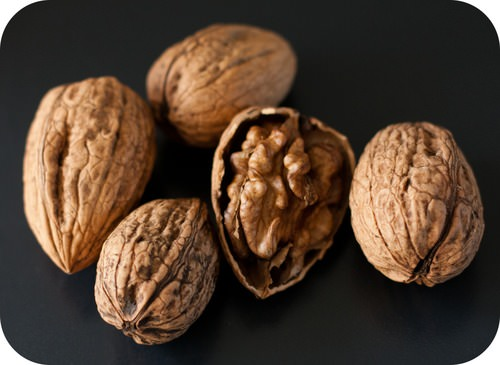# 5.27: Mass-Mass Stoichiometry

$$\newcommand{\vecs}{\overset { \rightharpoonup} {\mathbf{#1}} }$$ $$\newcommand{\vecd}{\overset{-\!-\!\rightharpoonup}{\vphantom{a}\smash {#1}}}$$$$\newcommand{\id}{\mathrm{id}}$$ $$\newcommand{\Span}{\mathrm{span}}$$ $$\newcommand{\kernel}{\mathrm{null}\,}$$ $$\newcommand{\range}{\mathrm{range}\,}$$ $$\newcommand{\RealPart}{\mathrm{Re}}$$ $$\newcommand{\ImaginaryPart}{\mathrm{Im}}$$ $$\newcommand{\Argument}{\mathrm{Arg}}$$ $$\newcommand{\norm}{\| #1 \|}$$ $$\newcommand{\inner}{\langle #1, #2 \rangle}$$ $$\newcommand{\Span}{\mathrm{span}}$$ $$\newcommand{\id}{\mathrm{id}}$$ $$\newcommand{\Span}{\mathrm{span}}$$ $$\newcommand{\kernel}{\mathrm{null}\,}$$ $$\newcommand{\range}{\mathrm{range}\,}$$ $$\newcommand{\RealPart}{\mathrm{Re}}$$ $$\newcommand{\ImaginaryPart}{\mathrm{Im}}$$ $$\newcommand{\Argument}{\mathrm{Arg}}$$ $$\newcommand{\norm}{\| #1 \|}$$ $$\newcommand{\inner}{\langle #1, #2 \rangle}$$ $$\newcommand{\Span}{\mathrm{span}}$$$$\newcommand{\AA}{\unicode[.8,0]{x212B}}$$Figure $$\PageIndex{1}$$ (Credit: Pauline Mak; Source: http://www.flickr.com/photos/__my__photos/5591677002/(opens in new window); License: CC ​​​​​​​by 2.0(opens in new window))

### How many walnuts are needed to equal 250 grams?

I want to send 250 grams of shelled walnuts to a friend (don't ask why- just go with the question). How many walnuts in shells do I need to buy? To figure this out, I need to know how much the shell of a walnut weighs (about $$40\%$$ of the total weight of the unshelled walnut). I can then calculate the mass of walnuts that will yield 250 grams of shelled walnuts, and then determine how many walnuts I need to buy.

## Mass to Mass Problems

Mass-mass calculations are the most practical of all mass-based stoichiometry problems. Moles cannot be measured directly, while the mass of any substance can generally be easily measured in the lab. This type of problem is three steps, and is a combination of the two previous types.

$\text{mass of given} \rightarrow \text{moles of given} \rightarrow \text{moles of unknown} \rightarrow \text{mass of unknown}\nonumber$

The mass of the given substance is converted into moles by use of the molar mass of that substance from the periodic table. Then, the moles of the given substance are converted into moles of the unknown by using the mole ratio from the balanced chemical equation. Finally, the moles of the unknown are converted to mass by use of its molar mass.

##### Example $$\PageIndex{1}$$: Mass-Mass Stoichiometry

Ammonium nitrate decomposes to dinitrogen monoxide and water, according to the following equation.

$\ce{NH_4NO_3} \left( s \right) \rightarrow \ce{N_2O} \left( g \right) + 2 \ce{H_2O} \left( l \right)\nonumber$

In a certain experiment, $$45.7 \: \text{g}$$ of ammonium nitrate is decomposed. Find the mass of each of the products formed.

###### Solution

Step 1: List the known quantities and plan the problem.

###### Known
• Given: $$45.7 \: \text{g} \: \ce{NH_4NO_3}$$
• $$1 \: \text{mol} \: \ce{NH_4NO_3} = 1 \: \text{mol} \: \ce{N_2O} = 2 \: \text{mol} \: \ce{H_2O}$$
• Molar mass of $$\ce{NH_4NO_3} = 80.06 \: \text{g/mol}$$
• Molar mass of $$\ce{N_2O} = 44.02 \: \text{g/mol}$$
• Molar mass of $$\ce{H_2O} = 18.02 \: \text{g/mol}$$
###### Unknown
• Mass $$\ce{N_2O} = ? \: \text{g}$$
• Mass $$\ce{H_2O} = ? \: \text{g}$$

Perform two separate three-step mass-mass calculations, as shown below.

$\text{g} \: \ce{NH_4NO_3} \rightarrow \text{mol} \: \ce{NH_4NO_3} \rightarrow \text{mol} \: \ce{N_2O} \rightarrow \text{g} \: \ce{N_2O}\nonumber$

$\text{g} \: \ce{NH_4NO_3} \rightarrow \text{mol} \: \ce{NH_4NO_3} \rightarrow \text{mol} \: \ce{H_2O} \rightarrow \text{g} \: \ce{H_2O}\nonumber$

###### Step 2: Solve.

$45.7 \: \text{g} \: \ce{NH_4NO_3} \times \frac{1 \: \text{mol} \: \ce{NH_4NO_3}}{80.06 \: \text{g} \: \ce{NH_4NO_3}} \times \frac{1 \: \text{mol} \: \ce{N_2O}}{1 \: \text{mol} \: \ce{NH_4NO_3}} \times \frac{44.02 \: \text{g} \: \ce{N_2O}}{1 \: \text{mol} \: \ce{N_2O}} = 25.1 \: \text{g} \: \ce{N_2O}\nonumber$

$45.7 \: \text{g} \: \ce{NH_4NO_3} \times \frac{1 \: \text{mol} \: \ce{NH_4NO_3}}{80.06 \: \text{g} \: \ce{NH_4NO_3}} \times \frac{2 \: \ce{H_2O}}{1 \: \text{mol} \: \ce{NH_4NO_3}} \times \frac{18.02 \: \text{g} \: \ce{H_2O}}{1 \: \text{mol} \: \ce{H_2O}} = 20.6 \: \text{g} \: \ce{H_2O}\nonumber$

The total mass of the two products is equal to the mass of ammonium nitrate which decomposed, demonstrating the law of conservation of mass. Each answer has three significant figures.

## Summary

• Mass-mass calculations involve converting the mass of a reactant to moles of reactant, then using mole ratios to determine moles of product which can then be converted to mass of product.

## Review

1. If matter is neither created nor destroyed, why can’t we just go directly from grams of reactant to grams of product?
2. Why is it important to get the subscripts correct in the formulas?
3. Why do the coefficients need to be correct?

5.27: Mass-Mass Stoichiometry is shared under a CC BY-NC license and was authored, remixed, and/or curated by LibreTexts.